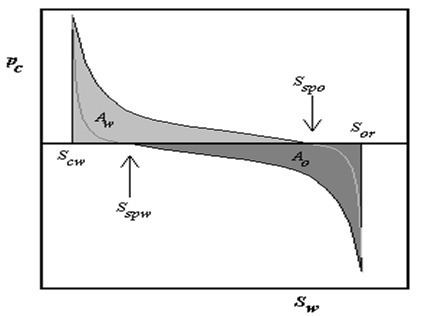# Amott test

Updated on
Covid-19The Amott test is one of the most widely used empirical wettability measurements for reservoir cores in petroleum engineering. The method combines two spontaneous imbibition measurements and two forced displacement measurements. This test defines two different indices: the Amott water index ( I w ) and the Amott oil index ( I o ).

## Amott–Harvey index

The two Amott indices are often combined to give the Amott–Harvey index. It is a number between -1 and 1 describing wettability of a rock in drainage processes. It is defined as:

A I = I w I o

These two indices are obtained from special core analysis (SCAL) experiments (porous plate or centrifuge) by plotting the capillary pressure curve as a function of the water saturation as shown on figure 1:

I w = S s p w S c w S o r S c w

with S s p w is the water saturation for a zero capillary pressure during the imbibition process, S c w is the irreducible water saturation and S o r is the residual oil saturation after imbibition.

I o = S o r S s p o S o r S c w

with S s p o is the oil saturation for a zero capillary pressure during the secondary drainage process, S c w is the irreducible water saturation and S o r is the residual non-wetting phase saturation after imbibition.

A rock is defined as:

• Water wet when the Amott–Harvey index is between 0.3 and 1,
• Weakly water wet when the Amott–Harvey index is between 0 and 0.3,
• Weakly oil wet when the Amott–Harvey index is between -0.3 and 0,
• Oil wet when the Amott–Harvey index is between -1 and -0.3.
• ## References

Similar Topics
The Greatest Question
Simon Bamford
Gillian Shephard
Topics